Next: Smoothness estimation Up: Theory Previous: Theory

## Statistical inference

The set of voxels exceeding some threshold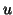comprises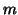clusters each of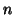voxels. The expectations of,and the total number of voxels above threshold,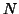, are related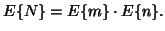(1)

For our purposes we can calculate all the necessary information knowing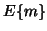and this can be approximated by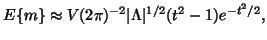(2)

whereis an estimate of the smoothness of the GRF,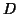the number of dimensions in the GRF and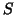is the number of in-brain voxels.

The probability of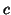clusters in this excursion set is approximated by a Poisson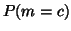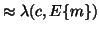(3)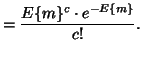(4)

The number of voxelscomprising a cluster is distributed according to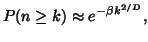(5)

where(6)

We can calculate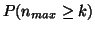by calculating the product of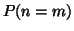and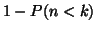summed over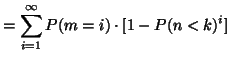(7)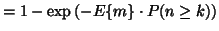(8)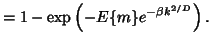(9)Next: Smoothness estimation Up: Theory Previous: Theory
David Flitney 2001-11-29